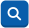【发布时间：2016年10月14日】

1.水资源费征收凭证库(AO电子账簿)，主要包括：科目编码、科目名称、凭证日期、摘要、凭证号、借方金额

2.水资源费征收台账，主要包括：单位名称、用水量

3.水资源费征收标准，按用水量每吨1.60元计征

1.生成水资源费实收表。

Sql语句：Select [凭证日期],[凭证号],[摘要],[借方金额] INTO 水资源费实收表 From [凭证库] WHERE [科目编码]='10203' AND [借方金额]>0

2.生成水资源费直接实收表。

Sql语句：Select * INTO [水资源费直接实收表] From [水资源费实收表] WHERE [摘要] LIKE '收%'

3.整理水资源费直接实收表

Sql语句：

1）在AO 2008版中使用的查询语句：

UPDATE 水资源费直接实收表 SET 摘要 =MID([摘要],2,len([摘要])-5)

——利用文本函数将摘要中开头的“收”与结尾的“水资源费”五个字去除，只保留缴款单位（此语句在AO 2011版显示为：“'MID' 不是可以识别的内置函数名称”，运行不了）

2）在AO 2011版中可以使用以下查询语句：

①先查看摘要中字符长度。

Select distinct len(摘要) From 水资源费直接实收表 where 摘要 like '收%' ORDER BY len(摘要) --显示字符长度为715

②在摘要截取出企业名称。

UPDATE 水资源费直接实收表 SET 摘要 =case

when len(摘要)=7 then substring(摘要,2,2)

when len(摘要)=8 then substring(摘要,2,3)

when len(摘要)=9 then substring(摘要,2,4)

when len(摘要)=10 then substring(摘要,2,5)

when len(摘要)=11 then substring(摘要,2,6)

when len(摘要)=12 then substring(摘要,2,7)

when len(摘要)=13 then substring(摘要,2,8)

when len(摘要)=14 then substring(摘要,2,9)

when len(摘要)=15 then substring(摘要,2,10)

end

——如摘要中字符长度为7，那么从第2位开始选2位截取出企业名称，依次类推

4.生成水资源费分单位全年实收表。

SELECT [摘要] AS 单位名称, Sum([借方金额]) AS 实收数 INTO 水资源费分单位全年实收表 FROM [水资源费直接实收表] GROUP BY [摘要]

5.生成水资源费应收表。

SELECT [应收单位名称], [用水量], [用水量]*1.60 AS 应收数 INTO 水资源费应收表 FROM [水资源费征收台账]

6.生成漏征水资源费表

SELECT a.[应收单位名称], a.[应收数], b.[单位名称], b.[实收数],a.[应收数] AS 差额 INTO 漏征水资源费表 FROM ([水资源费应收表] a LEFT JOIN [水资源费分单位全年实收表] b ON a.[应收单位名称]=b.[单位名称]) WHERE b.[单位名称] is null；

7.生成少征水资源费表

SELECT a.[应收单位名称], a.[应收数], b.[单位名称], b.[实收数],a.[应收数]-b.[实收数] AS 差额 INTO 少征水资源费表 FROM ([水资源费应收表] a JOIN [水资源费分单位全年实收表] b ON a.[应收单位名称]=b.[单位名称]) WHERE ABS(a.[应收数]-b.[实收数])>1

8. 汇总少征及漏征水资源费总额

1）生成少征及漏征水资源费表

Select * INTO 少征及漏征水资源费表 From [少征水资源费表] UNION Select * From [漏征水资源费表 ] ORDER BY [差额] DESC

2）计算出少征及漏征水资源费总额

Select COUNT([应收单位名称]) AS 应收单位数,SUM([应收数]) AS 应收数合计,COUNT([单位名称]) AS 实收单位数,SUM([实收数]) AS 实收数合计,SUM([差额]) AS 差额合计 From [少征及漏征水资源费表]

 【关闭】    【打印】